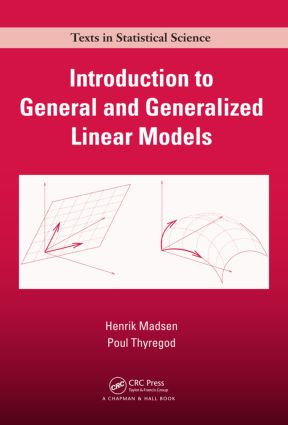Introduction to General and Generalized Linear Models

1st Edition

CRC Press

316 pages | 50 B/W Illus.

Purchasing Options:\$ = USD
Hardback: 9781420091557
pub: 2010-11-09
SAVE ~\$22.00
\$110.00
\$88.00
x
eBook (VitalSource) : 9780429111709
pub: 2010-11-09
from \$55.00

FREE Standard Shipping!

Description

Bridging the gap between theory and practice for modern statistical model building, Introduction to General and Generalized Linear Models presents likelihood-based techniques for statistical modelling using various types of data. Implementations using R are provided throughout the text, although other software packages are also discussed. Numerous examples show how the problems are solved with R.

After describing the necessary likelihood theory, the book covers both general and generalized linear models using the same likelihood-based methods. It presents the corresponding/parallel results for the general linear models first, since they are easier to understand and often more well known. The authors then explore random effects and mixed effects in a Gaussian context. They also introduce non-Gaussian hierarchical models that are members of the exponential family of distributions. Each chapter contains examples and guidelines for solving the problems via R.

Providing a flexible framework for data analysis and model building, this text focuses on the statistical methods and models that can help predict the expected value of an outcome, dependent, or response variable. It offers a sound introduction to general and generalized linear models using the popular and powerful likelihood techniques. Ancillary materials are available at www.imm.dtu.dk/~hm/GLM

Reviews

This book presents a well-structured introduction to both general linear models and generalized linear models. … I would recommend the book as a suitable text for senior undergraduate or postgraduate students studying statistics or a reference for researchers in areas of statistics and its applications.

—Shuangzhe Liu, International Statistical Review, 2012

This book is targeted to undergraduates in statistics but can be used by researchers as a reference manual as well. It is well written, easy to read and the discussion of the examples is clear. As a complement there is a collection of slides for an introductory course on general, generalized, and mixed effects models in the homepage cited in the preface of this book. This book has a good set of references … I recommend this book as one of the textbooks to be discussed in a course for model building.

—Clarice G.B. Demétrio, Biometrics, February 2012

Introduction

Examples of types of data

Motivating examples

A first view on the models

The Likelihood Principle

Introduction

Point estimation theory

The likelihood function

The score function

The information matrix

Alternative parameterizations of the likelihood

The maximum likelihood estimate (MLE)

Distribution of the ML estimator

Generalized loss-function and deviance

Quadratic approximation of the log-likelihood

Likelihood ratio tests

Successive testing in hypothesis chains

Dealing with nuisance parameters

General Linear Models

Introduction

The multivariate normal distribution

General linear models

Estimation of parameters

Likelihood ratio tests

Tests for model reduction

Collinearity

Inference on parameters in parameterized models

Model diagnostics: residuals and influence

Analysis of residuals

Representation of linear models

General linear models in R

Generalized Linear Models

Types of response variables

Exponential families of distributions

Generalized linear models

Maximum likelihood estimation

Likelihood ratio tests

Test for model reduction

Inference on individual parameters

Examples

Generalized linear models in R

Mixed Effects Models

Gaussian mixed effects model

One-way random effects model

More examples of hierarchical variation

General linear mixed effects models

Bayesian interpretations

Posterior distributions

Random effects for multivariate measurements

Hierarchical models in metrology

General mixed effects models

Laplace approximation

Mixed effects models in R

Hierarchical Models

Introduction, approaches to modelling of overdispersion

Hierarchical Poisson gamma model

Conjugate prior distributions

Examples of one-way random effects models

Hierarchical generalized linear models

Real-Life Inspired Problems

Dioxin emission

Depreciation of used cars

Young fish in the North Sea

Traffic accidents

Mortality of snails

Appendix A: Supplement on the Law of Error Propagation

Appendix B: Some Probability Distributions

Appendix C: List of Symbols

Bibliography

Index

Problems appear at the end of each chapter.

Copenhagen, , Denmark